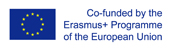This project has been funded with support from the European Commission.
This web site reflects the views only of the author, and the Commission cannot be held responsible for any use which may be made of the information contained therein.

Select language   >   IT EN RO PO LT
Login

### Exponential Growth

Subject taught
- Math

Type of Product
- Multiple choice questionnaire
- Web Site/Portal

Language Skills Developed
- Interaction
- Listening
- Reading
- Speaking
- Writing

Transferable/Scientific Skills Developed
Students will be able to apply the knowledge in real life: The price of the accomodation The meaning of the investments

Description
The aim of the mathematics – to enable students to develop mathematical competence, that is abilities and attitudes, to get acquainted with the world, to describe it with mathematical models, to use mathematical methods in solving practical and theoretical problems in various fields of science; To get students interested in the subject of mathematics and its application, to promote personal initiative with the help of individual development in mathematics;
To expand and deepen thematic knowledge of the material;
To link to life situations providing a broader understanding of life;
To develop logical thinking, creativity, imagination and fantasy;
The students will expand the mathematical vocabulary, will be able to explain concepts in English.

Exponential growth occurs when the number of some entities increases exponentially over time. The exponential mathematical function of growth is the one in which the numbers increase while the time passes. Examples of exponential growth can be seen in bacterial populations which divide very quickly. For example, Salmonella enterica serovario Typhimurium bacteria have been extensively studied and found to have a delayed phase during which they prepare to enter an exponential growth model. Bacteria will excrete and the population will will grow exponentially untill more no more nutrients remain. Therefore, it is important for students to be able to explain what the exponential growth is, its importance, and its application in real life.
Tasks are assigned to the students of the 11-12 forms.
The time required for using the teaching material with students is one academic hour (45 minutes).
First of all, students have an opportunity to examine the examples provided, to get acquainted with the „Half Life of Medicine“ tool, which will allow to better understand the conceipt of exponential growth.
When examining the exponential growth, it is important to get acquanted with the exponential decay as well. Difficulty is the interpratation of mathematical perception in the context of exponential growth.

Comments
Strength – the opportunity to understand and analyze the importance of exponential growth in real life, to discover interdisciplinary connections. Weakness – the explanation of mathematical perception of exponential growth to lower grade students. Scientific reliability: For the representatives of the exact sciences exponential growth will sound banal: the exponential. What could be more boring in maths than the exponent? Let‘s integrate the exponent, what will we get? The exponent! Let‘s count its derivative, what do we see? Again – the „immortal“ exponent... The exponent is one of the most boring functions for theorists, but it is surprisingly common in the world around us: in biology, physics, chemistry, and elsewhere. Exponential growth can also be described mathematically using equations containing the exponent. Exponential growth fates a rapid change in a number which coefficient is always positive and bigger than 1. Exponential growth is when numbers increase rapidly exponentially. An example of exponential growth is the rapid growth of a bacterial population. The self-directed learning method will be applied to the examination of the topic. This method will allow each student to examine the field of science which he/she likes, in which there is importance of the exponential growth.